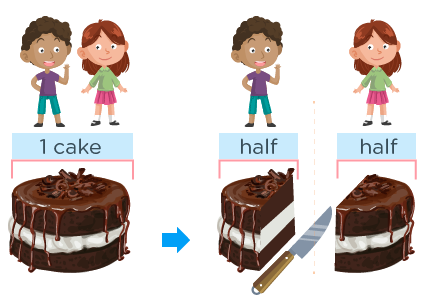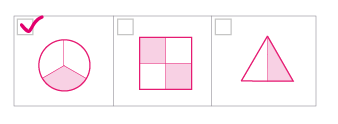# Fraction worksheets for kindergarten: printable and fun activities

Are you looking for engaging and educational fraction worksheets for your kindergarten students? Do you want to help them develop a solid foundation in math and fractions? If so, you are in the right place!

In this resource, we will share some amazing free printable and fun fraction worksheets and activities you can use in your classroom or at home. These worksheets and activities are designed to introduce fractions to kindergarten students in a fun and interactive way.

• They cover different difficulty levels and skills, such as identifying fractions, comparing fractions, coloring fractions, and more.

You will also find some tips and tricks for teaching fractions effectively and creatively and some fun games and puzzles to reinforce fraction concepts and practice.

## What are fractions, and why are they important?

Apart from learning and understanding what fractions are, finding out why they are important in math and our daily lives is also necessary.

Fractions are a way of representing parts of a whole. For example, if you have a pizza and cut it into four equal slices, each slice is one-fourth or one-quarter of the pizza.

Fractions help us measure and compare quantities that are not whole numbers, such as half a cup of milk, three-quarters of an hour, or two-thirds of a candy bar.

Fractions are essential because they are everywhere in our daily life. We use them to tell time, measure ingredients for cooking, divide things equally among people, describe shapes and patterns, and more.

In addition, fractions help us develop logical thinking and problem-solving skills. They prepare us for more advanced math topics like decimals, percentages, ratios, and proportions.

• ### How to introduce fractions to kindergarten students with fun activities?

The best way to introduce fractions to kindergarten students is to use concrete objects and manipulatives they can see, touch, and explore. For example, you can use:

• Food items include pizzas, pies, cookies, cakes, sandwiches, etc.
• Shapes, such as circles, squares, rectangles, triangles, etc.
• Paper or cardboard cut-outs of shapes or food items
• Fraction tiles or strips
• Fraction circles or bars
• Fraction spinners or dice
• Fraction dominoes or cards

You can use these objects and manipulatives to show your students how to:

• Divide a whole into equal parts
• Name the parts using fraction words and symbols
• Identify the numerator (the number of parts) and the denominator (the total number of parts) in a fraction
• Compare fractions with the same or different denominators using words like equal, more than, less than, etc.
• Find equivalent fractions (fractions that have the same value but different names) by using models or pictures
• Add or subtract simple fractions with the same denominator by counting the parts
• ### Fraction worksheets for different levels of difficulty and skills

To help your students practice and master fractions, you can use the worksheet we created for you. They are printable and easy to use. You can choose from different difficulty levels and skills according to your student's needs and abilities.

Here are some examples of the fraction worksheets that you can find in this resource:

• Identifying fractions: These worksheets help your students recognize fractions using pictures or models. They have to name the fraction shown or match it with the correct word or symbol.
• Comparing fractions: These worksheets help your students compare fractions using pictures or models. They must use words like equal, more than, less than, etc., to compare two or more fractions.
• Coloring fractions: These worksheets help your students practice coloring fractions using pictures or models. They have to color the given fraction of a shape or a group of objects.
• Cutting fractions: These worksheets help your students practice cutting fractions using paper or cardboard cut-outs. They have to cut out the given fraction of a shape or a food item.
• ### Tips and tricks for teaching fractions effectively and creatively

To make your fraction lessons more effective and creative, you can use these tips and tricks that we acquired from our experience as kindergarten tutors:

• Use real-life examples and contexts to make fractions relevant and meaningful for your students. For example, you can use food items that they like or are familiar with, such as pizzas, pies, cookies, cakes, sandwiches, etc.
• Use different types of objects and manipulatives to show fractions in various ways. For example, you can use shapes, paper cut-outs, fraction tiles or strips, fraction circles or bars, fraction spinners or dice, fraction dominoes or cards, etc.
• Use games and puzzles to make fractions fun and engaging for your students. For example, you can use bingo, memory, matching, sorting, dominoes, puzzles, etc.

For example, you can use this song:

" One whole is two halves,
Two halves make one whole.
One whole is four quarters,
Four quarters make one whole.
One whole is three thirds,
Three thirds make one whole.
Fractions are easy,
Fractions are fun!
We love learning fractions,
We're number one! "

• Use stories and books to introduce fraction concepts and vocabulary. For example, you can use this book:

The Doorbell Rang by Pat Hutchins

This is a story about two children with 12 cookies to share equally. But every time the doorbell rings, more friends arrive, and they have to divide the cookies into smaller and smaller parts. This book helps your students understand how fractions can divide things equally among people.

• ### Fun games and puzzles to reinforce fraction concepts and practice

To reinforce fraction concepts and practice, you can use these fun games and puzzles we created for you. They are printable and easy to use. You can play them with your students in small groups or pairs.

Here are some examples of the games and puzzles that you can find in this article:

• Fraction bingo: This is a game where your students have to match fraction pictures or models with fraction words or symbols on their bingo cards. The first one to get five in a row wins.
• Fraction memory: This is a game where your students have to match pairs of cards that show equivalent fractions using pictures or models. The one with the most pairs wins.
• Fraction matching: This is a game where your students have to match cards that show fractions with the same value but different names using pictures or models. The one with the most matches wins.
• Fraction sorting: This is a game where your students can sort cards that show fractions into categories based on their size (more than half, less than half, or equal to half) using pictures or models. The one who sorts all the cards correctly wins.
• Fraction dominoes: This is a game where your students have to connect dominoes that show equivalent fractions using pictures or models. The one who uses all their dominoes first wins.
• Fraction puzzles: These are puzzles where your students have to complete pictures that show fractions using pieces of fraction parts. The one who completes all the puzzles correctly wins.
• ### Important facts about fraction for Kinders

Given that fractions represent equal parts of a whole, our free fraction worksheet pdf are made up of simple shapes divided in equal and unequal fractional parts.

This beginning fractional concept is aimed at helping kids to easily know and thus identify shapes with equal parts only.

As they do this, they are actually building an excellent foundation to a perfect understanding of fractions. Equally important, our Fraction vocabulary for kindergarten, consist of a marvelous approach which will help kids to have an easy mastery of fractional words such as halves, thirds and fourths

Fractions is one of kid’s first experiences beyond the knowledge of addition and subtraction. Notwithstanding, this printable fraction worksheets for kindergarten will help kids overcome the greatest challenge of manipulating fractions, and as well understand how numbers interact. Consequently, they’ll grab the concept of division at their fingertips.

### Best fun fraction activities for kinders – foundational block for advanced math

Ooops, it’s all about fun and easy math from kindergarten to higher heights. As parents engage their kids in this best fun fraction activities for kinders, they are in essence laying a foundational block for advanced math.

Being fluent in fraction vocabulary for kindergarten enhances and prepares kids for huge success in higher math’s concepts, such as algebra, geometry, highest common factor, lowest common multiple and many others.

“Hey kids, do you even realize that you use fractions in your daily lives? Not sure I think” just imagine that you want to equally share 1 cake with your friend. An equal share will therefore mean that each person will obtain only half.This fun fraction vocabulary for kindergarten activity builds confidence in kids and a quest for further learning. Given the exercise below, we’ll therefore determine which shapes are halves, thirds or fourths.

### Example: halves, thirds and fourths - Which shape shows thirds?

It is very easy to understand the concept of halves, thirds and fourths. Considering the fact that they are very basic concepts, kids simply need to have in mind that;

• halves signify two (2) parts
• Thirds signify three (3) parts
• Fourths signify four (4) parts

So following our question which shape shows thirds? We’ll look for the shape that is divided into 3 parts.We have placed a tick on the first shape, because it has been divided into 3 parts, thus “thirds”.

Wow! Just so awesome and very simple.

Thank you very much for choosing to share the MathSkills4Kids.com links with your loved ones!

I hope you enjoyed this article and found it helpful in teaching fractions to your kindergarten students. With these fraction worksheets, tips, tricks, games, and puzzles, you’ll agree that fractions are essential, fun, and easy to learn.

If you liked this resource, please share it with your friends and colleagues who might also be interested in teaching fractions to their kindergarten students.

Thank you for reading, and happy teaching!